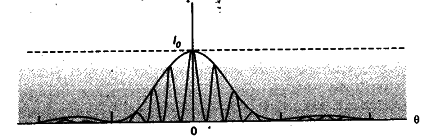# Explain by drawing a suitable diagram that the interference pattern in a double slit is actually a superposition of single slit diffraction from each slit

Explain by drawing a suitable diagram that the interference pattern in a double slit is actually a superposition of single slit diffraction from each slit.Write two basic features which distinguish the interference pattern from those seen in a coherently illuminated single slitThe diagram, given here, shows several fringes, due to double slit interference/contained’ in a broad diffraction peak. When the separation between the slits is large compared to their width, the diffraction pattern becomes very flat and we observe the two slit interference pattern. 1
[Note : The students may be awarded 1 mark even if they just draw the diagram.Two basic features :
(i) The interference pattern has a number of equally
spaced bright and dark bands while diffraction pattern has a central bright maximum which is twice as wide as the other maxima.
(ii) Interference pattern is the superimposition of two waves slits originating from two narrow slits. The diffraction pattern is a superposition of a continuous family of waves originating from each point on a single slit.
(iii) For a single slit of width V the first null of diffraction pattern occurs at an angle of Lanbda/A. At the
same angle of Lambda/a, we get a maxima for two narrow slits separated by a distance a.

Are you sure you are correct in saying as d increases diffraction pattern will become flat

Isn’t that ‘a’ that will affect the diffraction pattern